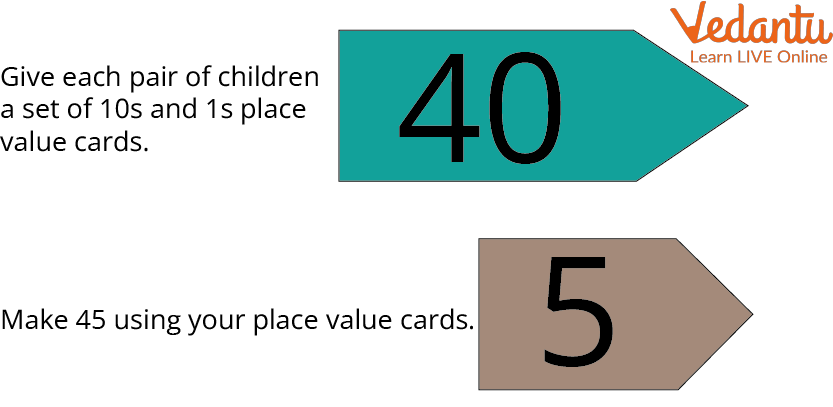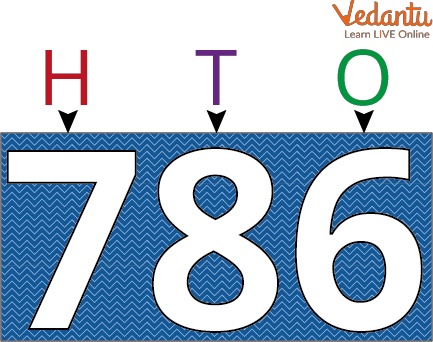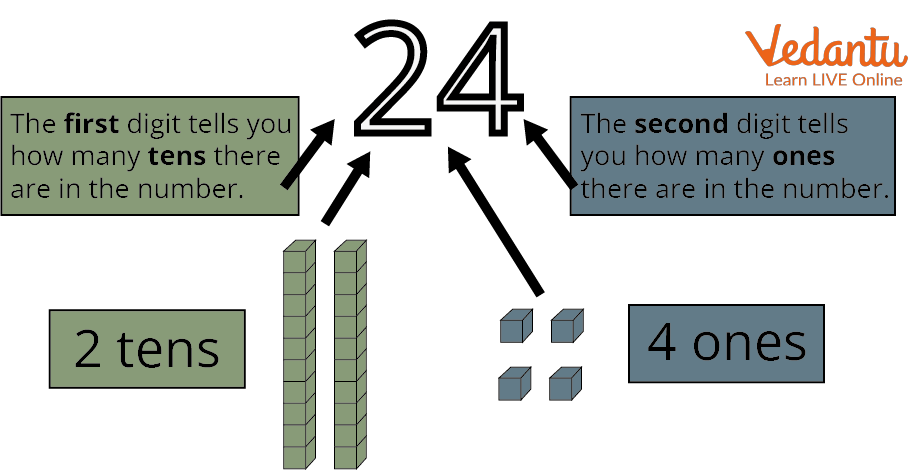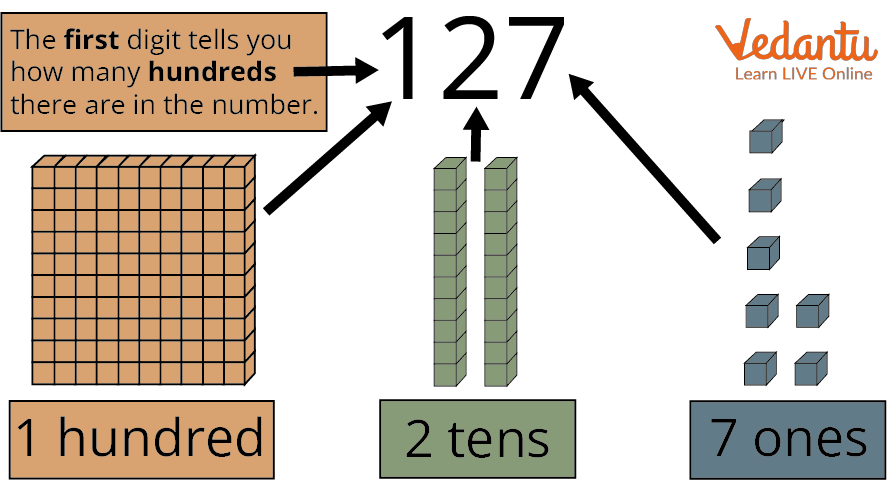Courses
Courses for Kids
Free study material
Free LIVE classes
More

# Ones, Tens and Hundreds## Introduction to Place Value of the Number

Understanding place value is crucial once we begin learning about the number system. One digit, two, three, and so on are all possible for a number. In the number system, each digit of a number has a unique position and value.

In Mathematics, the place values of the digits that follow the decimal point are designated as tenths and hundredths. Moving from right to left, the place values of a number's digits are ones, tens, and hundreds. In this article, we will study how to determine the place value for a number.

## How to Determine the Place Value?

We have already explained that each digit in a number has a unique place and value. We'll start by providing a basic example of a two-digit number to explain this: 45 further

This number has two digits, as can be seen. Beginning on the right side, please. "4" is the first number. This is at one's location. Hence its value is 5. "5" is the second number from the right. Since it is in the tens place, its value is 40. Instead of saying that 45 is composed of 4 and 5, we might say that it is composed of 40 and 5. Another way to put it is that it is composed of 4 tens and 5 ones.Tens and Ones

Now, if the larger number includes three digits, we will start at the right and assign a value to each position. We'll further explain this with a three-digit number example. Let's say that we have the number 786.

Once more, we'll start on the right. One is represented by the initial digit, "6," which has the value 6. The value of the second "8" digit, which contains ten places, is 80. The value of the third "7" digit, which is in hundreds, is 700.

Instead of saying that the number is composed of 1, 2, and 3, we might say that it is composed of 700, 80, and 6. We can also see from the diagram below that it is composed of 6 ones, 8 tens, and 7 hundred.Ones Tens Hundred

## Examples Showing the Place Values of a Number in One's Tens Hundred

Suppose we have 2-digit numbers; let’s determine their place value.

1. With the first number, 24, we shall begin. There are two digits. In this illustration, the value of 4 is present at one's position. The value of 2 in the tens place is twenty. So, when 20 and 4 are added together, we get 24.Place value of 24

1. Additionally, the number 44 has two digits. 4 is at one's position, starting from the right, and its value is 4. The following four are in the tens position and have a value of 40. So we get 44 when we add 40 and 4.

2. The last illustration uses the number 89. This two-digit number is worth nine and has nine at the one position. Its value is 80, and it has an 8 at the tens position. We obtain 89 by adding 80 and 9.

Let's now determine the place value (hundreds, tens and units) of the three-digit numbers below:

1. The first number is 127- The first number is worth 7, and it has a 7 at the position of the one. Its value is 20, and it has a 2 at the tens position. At hundreds, it has 1 whose value is 100. Thus, we can say that by joining 100, 20, and 7, we get 127.Place Value of 127

1. The second number is 240- At one place, the value given is 0. Its value is 40, and it has 4 at ten places. Its value is 200, and it has 2 in the hundreds position. We obtain 240 by adding 200 and 40.

2. The third number we can use is 609. The third number in one place is 9. Its value is 0, and it has 0 at 10 places. Its value is 600, and it has 6 in the hundreds position. Thus, by adding 600 and 9, we obtain 609.

## Worksheet for Practise

Given below are a few questions which you need to solve and test your learning so far.

Q1. Write how many hundreds, tens and units are there in the given numbers. Here units are termed as ones.

1. 21

2. 34

3. 40

4. 12

5. 87

6. 457

7. 768

Ans: 1. 20, 1

2. 30, 4

3. 40,0

4. 10, 2

5. 80, 7

6. 400, 50, 7

7. 700, 60, 8

Q2. Write them as decimals-

1. Five ones and one-tenths

2. Forty and seven-tenth

3. Twenty and five- tenth

Ans: 1. 5.10

2. 40.7

3. 20.5

Q3. How to write 2 rupees in paisa?

Ans: 200 paise.

Q4. Find the place value of the 124.

Ans: The first number has 4 at one's place, and its value is 4. It has 2 at tens place, and its value is 20. It has 1 at hundreds number place, and its value is 100.

## Summary

Thus, it is crucial to comprehend the place value to understand the meaning of a number fully. If we learn about place values, we can use numbers more efficiently. Students can view the value of digits, integers, and decimals in our base ten number system using a place value chart. The idea of place value in mathematics is crucial because it determines the value of each digit in a number based on where it is in the number.

Last updated date: 24th Sep 2023
Total views: 109.2k
Views today: 1.09k

## FAQs on Ones, Tens and Hundreds

1. What is the place value of a number, and why is it important to learn it?

One digit, two digits, three digits, and so on are all possible for a number. In the number system, each digit of a number has a unique position and value. This place is known as the place value of the number.  It is crucial to comprehend the idea of place value to understand the meaning of a number fully. If we learn about place value, we can use numbers more efficiently. For example- in the number 45, the place value will be 4 tens and 5 ones.

2. Write how many hundreds, tens and ones are present in the given numbers- 240 and 609.

Hundreds, tens and ones are present in the given numbers are:

1. 240 - The value of the last number present in one's place is zero. Its value is 40, and it has 4 at ten places. Its value is 200, and it has 2 in the hundreds position. We obtain 240 by adding 200 and 40.

2. 609 - The third number in one place is nine. Its value is 0, and it has 0 at tens of places. Its value is 600, and it has 6 in the hundreds position. Thus, by adding 600 and 9, we obtain 609.

3. What is the concept of tens and ones?

In a two-digit number, the value of the digit depends on its position in that number. At one's place, the digit at the extreme right is known to be like one's, whereas the digit placed at the leftmost is known to be at ten's.